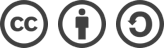## SDUT 3548 疯狂的小金（贪心）解题报告

2016-06-08 977 次浏览

## 题面

Time Limit: 1000MS Memory limit: 65536K

3 3
1 2 3
2 1
3 2
4 3

6

“师创杯”山东理工大学第八届ACM程序设计竞赛

## 解题思路

#include <cstdio>
#include <algorithm>
#include <functional>
#include <queue>
using namespace std;

struct info {
int v, w;
bool operator < (const info & cmp) const {
return v > cmp.v;
}
} a;

int main(int argc, char const *argv[]) {
int n, m, d;
while(~ scanf("%d %d", &n, &m)) {
for(int i=0; i<n; ++i) {
scanf("%d", &d[i]);
}
for(int i=0; i<m; ++i) {
scanf("%d %d", &a[i].v, &a[i].w);
}
if(n > m) {
printf("No Solution\n");
continue;
}
sort(d, d+n, greater<int>());
sort(a, a+m);
priority_queue<int, vector<int>, greater<int> > q;
bool ok = true;
long long ans = 0;
for(int i=0, j=0; i<n; ++i) {
for(; j<m; ++j) {    //把剩余弓箭中可以射杀该鸟的箭都加入到队列中
if(a[j].v >= d[i]) q.push(a[j].w);
else break;
}
if(!q.empty()) {
ans += q.top();    //用队首的箭（即价值最小的箭）射杀该鸟
q.pop();
}
else {    //如果此鸟无箭可杀，则无解
ok = false;
break;
}
}
if(ok) printf("%lld\n", ans);
else printf("No Solution\n");
}

return 0;
}bLue 创作，采用 知识共享署名 3.0，可自由转载、引用，但需署名作者且注明文章出处。